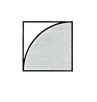Lesson 15 Circle functions

# Episode 1

Reasoning Resources: 4 xX 4 grid on board or OHT, circle drawn on 4 X 4 grid
Whole class introduction
Focusing pupils' attention on areas of circles drawn on grid.

Approaching the notion of 'area as a square-function' through visual or practical experience of constructing a square on the radius.

Comparing the area of the square on the radius to the area of a quarter circle with that radius. The quarter circle becomes an intermediate calculating unit, keeping the sense of size of the circle and its relation to the radius.Show a 4 by 4 grid, with the central cross heavy. How many unit squares in this quarter? In each quarter? And how many altogether? How could you find out how many squares there are in this quarter, without counting? Show the circle. What is its radius? Pupils should identify the centre of the circle, the fact that it is touching the sides, that it has a radius of 2 and a diameter of 4, that its area is definitely less than the area of the square around it. You may move to 6 X 6 grid, and 8 X 8 grids with similar discussion.Shade one quadrant of the circle on the board and ask for an estimate of its area: How many squares are in this part of the circle? Pupils’ answers could vary according to their recognition that they can use parts of squares, How many in the whole circle Pupils may give different answers by multiplying by four, e.g.’A bit more than ten."“About 12.’

With some classes you may wish to cut quarter circles out of squares and show how the trimmed parts from three squares nearly cover one of the quadrants. Give out Worksheet 1, asking: Does the same thing happen with different size circles?

.
Pair and small group work
More than one example is needed to ensure generalisation.

Constructing the coverage of the circle as a multiplicative relation, intuitively approaching the pi ratio through a '3 and a bit' phrase.

Use of approximation.

Inverse relation is possible with some classes, using an approximation of 3 for pi, and for a square root.
Pupils complete the square on the radius with a circle of radius 5. You may want to have a brief class discussion after question 4, to establish that the area of a circle is slightly more than three times the area of the square on the radius. In some classes, suggest using the approximate value of 3 for the ratio of the area of the circle to the square of its radius, with an understanding that this is a slight underestimate. In some other classes pupils may be able to use the value 3.1 or insist on using the calculator Constructing the coverage and rounding etc.
Whole class sharing and discussion
Empowering pupils to look at the world in mathematical relational terms, without mathematics being reduced to numeric accuracy. In final discussion emphasise the two steps of calculating the square of any number and the estimation of the area. Remind pupils of the circumference to radius ratio used in TM14: Tents. Let the pupils consider the use of values as an approximation in solving inverse problems, Use of approximation. from area to radius. Dividing by 3, then square rooting, is ‘doing the opposite operations, last step first! and gives a reasonable approximation. For example, to find the radius for a circle of area 100 units, we can first think of ‘roughly 30’ as area of the square on the radius, and therefore about 5.5 (or ‘something between 5 and 6’) as the length of the radius. The two- step approximation involved in finding the radius of a circle of a given area should be approached even with most classes. This could be made into a mental mathematics exercise, the results of which can be checked on the calculator. Approximation keeps the sense of size of numbers and is necessary for checking whether an answer on the calculator is reasonable.